The FREQ Procedure

Common Risk Difference

PROC FREQ provides Mantel-Haenszel and summary score estimates for the common risk (proportion) difference for multiway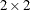tables. PROC FREQ also provides stratified Newcombe confidence limits for the common risk difference.

Mantel-Haenszel Estimate

PROC FREQ computes the Mantel-Haenszel (Mantel and Haenszel 1959) estimate of the common risk difference as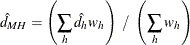where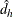is the risk difference in stratum h and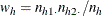The column 1 risk difference in stratum h is computed as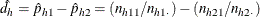where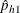is the proportion of row 1 observations that are classified in column 1 and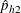is the proportion or row 2 observations that are classified in column 1. The column 2 risk is computed in the same way. For more information, see Agresti (2013, p. 231).

PROC FREQ computes the variance of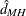(Sato 1989) as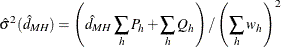where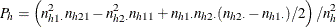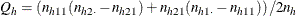The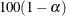% confidence limits for the common risk difference are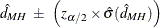Summary Score Estimate

PROC FREQ computes the summary score estimate of the common risk difference as described in Agresti (2013, p. 231). This estimate is computed from Miettinen-Nurminen (score) confidence limits for the stratum risk differences. For more information, see the section "Miettinen-Nurminen (Score) Confidence Limits." The score confidence interval for the risk difference in stratum h can be expressed as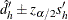, where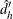is the midpoint of the score confidence interval and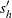is the width of the confidence interval divided by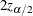. The summary score estimate of the common risk difference is computed as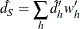where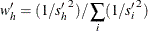The variance of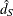is computed as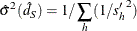The% summary score confidence limits for the common risk difference are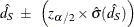Stratified Newcombe Confidence Limits

PROC FREQ computes stratified Newcombe confidence limits for the common risk (proportion) difference by using the method of Yan and Su (2010). The stratified Newcombe confidence limits are constructed from stratified Wilson confidence limits for the common (overall) row proportions.

PROC FREQ first computes individual Wilson confidence limits for the row proportions in eachtable (stratum), as described in the section Wilson (Score) Confidence Limits. These stratum Wilson confidence limits are then combined to form stratified Wilson confidence limits for the overall row proportions by using Mantel-Haenszel weights, where the Mantel-Haenszel weight for stratum h isThe confidence levels of the stratum Wilson confidence limits are chosen so that the overall confidence coefficient (for the stratified Wilson confidence limits) is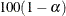% (Yan and Su 2010).

Denote the lower and upper stratified Wilson score confidence limits for the common row 1 proportion as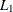and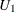, respectively, and denote the lower and upper stratified Wilson confidence limits for the common row 2 proportion as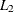and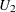, respectively. The% stratified Newcombe confidence limits for the common risk (proportion) difference are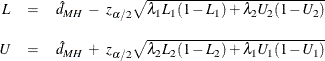whereis the Mantel-Haenszel estimate of the common risk difference and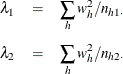When there is a single stratum, the stratified Newcombe confidence interval is equivalent to the (unstratified) Newcombe confidence interval. For more information, see the subsection "Newcombe Confidence Limits" in the section Confidence Limits for the Risk Difference. See also Kim and Won (2013).